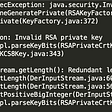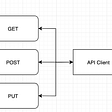# Euler Theorem in its core!

## In cryptography, there exists Euler Theorem which is based on Euler Totient Function which I already covered in my previous blog. Euler Theorem is also the base for Fermat’s Theorem & some other algorithms in Cryptography! This blog aims to explain the Euler Theorem at its core.

I would suggest going through my blog on Euler Totient Function before reading this one because the Euler Theorem is completely based on that. Therefore for better understanding, first read my previous blog on Euler Totient Function, if you haven’t already.

Euler has established his name in multiple fields by providing his great research work in multiple fields to this world. This theorem represents one of his amazing work in the field of Cryptography.

# Definition & Significance of the Euler Theorem!

It states that if there are 2 coprime numbers lets’ say p & q, then

where phi(q) is the Euler Totient Function.

The simplified equation for the above equation is:

Euler Theorem deals with the concept of prime numbers, modulus/remainder, & congruency. It aims to provide a concept where coprime numbers can be correlated somehow to provide a value that can be used later as a hash value or for encryption key in cryptography.

# Solved Example of the Euler Theorem!

Let us take 2 numbers 15 & 7, as they are coprime to each other. Let p = 15 & q = 7.

Since, 1mod(7) = 1, that is why mentioned in the above image.

Now, lets verify the LHS, phi(7) = 6, which transforms the LHS of the above equation as shown below:

where 15 ^ 6 = 11390625 => (15 ^ 6) Mod (7)= (11390625) Mod(7) = 1

As it comes 1, & also 1 mod(7) is also 1, therefore, LHS = RHS, & hence the theorem is verified.

I hope my article explains each and everything related to the topic with all the deep concepts and explanations. Thank you so much for investing your time in reading my blog & boosting your knowledge. If you like my work, then I request you to give an applaud to this blog!

--

--

--

## More from Analytics Vidhya

Analytics Vidhya is a community of Analytics and Data Science professionals. We are building the next-gen data science ecosystem https://www.analyticsvidhya.com

## Life of Lambda Labs with Justin## Migration from Asp.Net Core 2.2 to 3.1 — Real project## How To Get Text Of An Element In Selenium?## How to resolve RSA private key failed to load from pkcs12## Testing With OpenAPI## CCNA Routing and Switching 200–125 Certification: A Perfect Guide Summary.## Microsoft AppInsights or Revulytics Usage Intelligence?## CS 371p Spring 2022: Guan Lin Wee (Week 9)## Harshit Dawar

Big Data Enthusiast, have a demonstrated history of delivering large and complex projects. Interested in working in the field of AI and Data Science.

## More from Medium